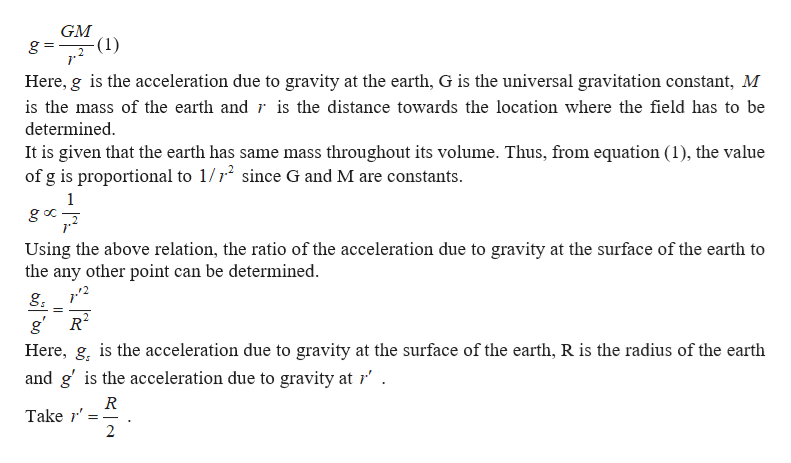# If Earth were of uniform density( same mass/volume throughout), what would the value of g be inside Earth at half its radius?

Question
2 views

If Earth were of uniform density( same mass/volume throughout), what would the value of g be inside Earth at half its radius?

check_circle

Step 1

The equation for the acceleration...help_outlineImage TranscriptioncloseGM g =- -(1) .2 Here, g is the acceleration due to gravity at the earth, G is the universal gravitation constant, M is the mass of the earth and r is the distance towards the location where the field has to be determined. It is given that the earth has same mass throughout its volume. Thus, from equation (1), the value of g is proportional to 1/1 since G and M are constants. ga Using the above relation, the ratio of the acceleration due to gravity at the surface of the earth to the any other point can be determined. g. 2. R g' Here, g, is the acceleration due to gravity at the surface of the earth, R is the radius of the earth and g' is the acceleration due to gravity at 1'. R Take r' fullscreen

### Want to see the full answer?

See Solution

#### Want to see this answer and more?

Solutions are written by subject experts who are available 24/7. Questions are typically answered within 1 hour.*

See Solution
*Response times may vary by subject and question.
Tagged in

### Gravitation